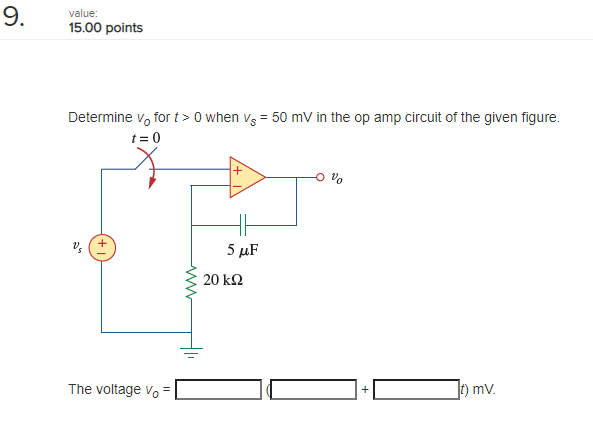# Question 9. value: 15.00 points Determine vo fort > O when V3 = 50 mV in the op amp circuit of the given figure. t = 0 us 5 20 ΚΩ The voltage vo + 10) mv.VHS4OM The Asker · Electrical EngineeringTranscribed Image Text: 9. value: 15.00 points Determine vo fort > O when V3 = 50 mV in the op amp circuit of the given figure. t = 0 us 5 20 ΚΩ The voltage vo + 10) mv.
More
Transcribed Image Text: 9. value: 15.00 points Determine vo fort > O when V3 = 50 mV in the op amp circuit of the given figure. t = 0 us 5 20 ΚΩ The voltage vo + 10) mv.